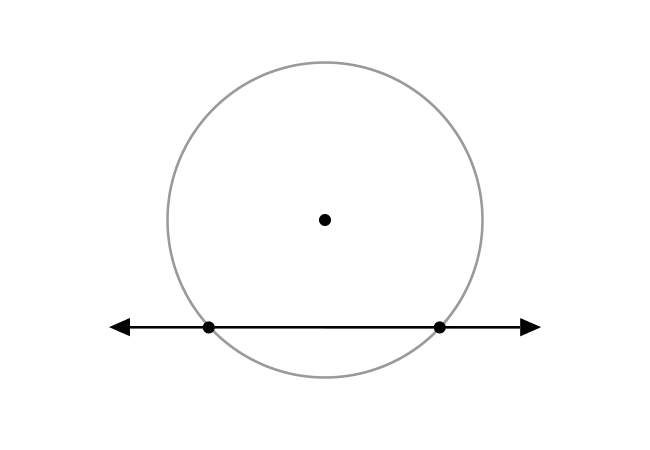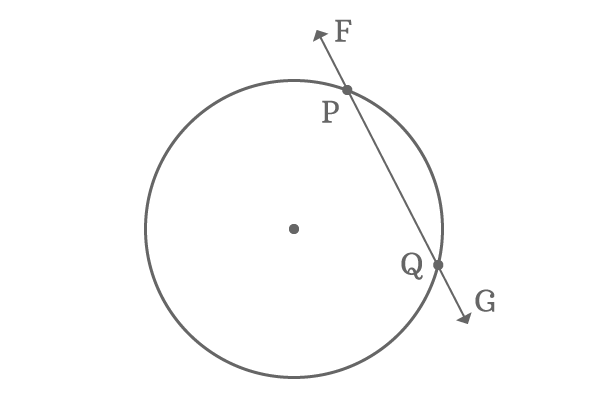# Secant of a Circle

A straight line that intersects a circle at any two points is called the secant line to a circle.

## IntroductionThe straight lines are often intersect the circles for some reasons in geometric mathematics. In some cases, the straight lines enter into the interior of the circles. In this case, a straight line can possibly intersect any circle at a maximum of two different points and the straight line is called the secant line to a circle.

When a straight line intersects a circle at two distinct points, the two points of the secant line lie on the circle.

### Example$P$ and $Q$ are any two points on the circumference of a circle.

$\overleftrightarrow{FG}$ is a straight line and it intersects the circle at two points $P$ and $Q$. So, the straight line $\overleftrightarrow{FG}$ is known as a secant of the circle at points $P$ and $Q$.

Latest Math Topics
Jun 26, 2023
Jun 23, 2023

###### Math Questions

The math problems with solutions to learn how to solve a problem.

Learn solutions

Practice now

###### Math Videos

The math videos tutorials with visual graphics to learn every concept.

Watch now

###### Subscribe us

Get the latest math updates from the Math Doubts by subscribing us.